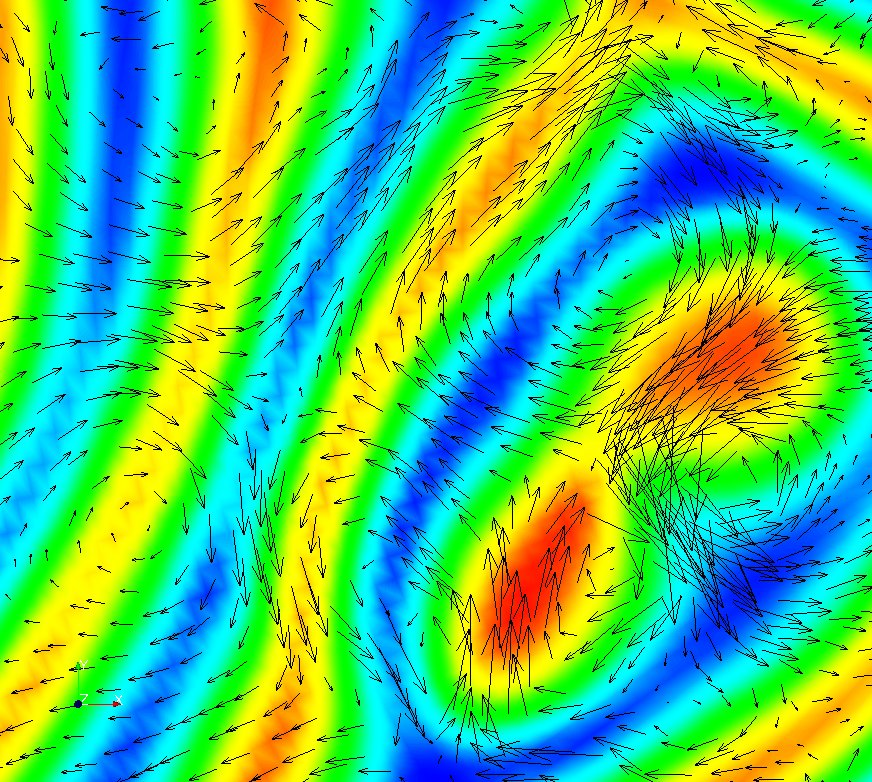# Simulations and Experiments

Description: A model of Earth's core, basically a spherical annulus of liquid sodium between a solid inner sphere (20 cm diameter) and a spherical outer container (60 cm). We can independently drive the two spheres to rotate, and we can apply dc external magnetic fields with a pair of magnets. Lately we've been studying inertial waves (a long-known phenomenon in rotating fluids) in the apparatus. What we actually measure is the oscillating magnetic field induced by the presence of the waves. Though the induction itself is linear, the excitation and amplification of the waves is not, and probably depends on instabilities. We've been able to show that the induction patterns are consistent with the induction due to specific (known) inertial wave velocity fields in a sphere. A spectrogram of the signal coming from one of our probes. For this data, the outer sphere is held at 30 Hz and the inner sphere rotation rate is varying along the horizontal axis. The vertical axis is signal frequency, and the color indicates spectral power at that frequency. Red areas have about 100x more power than blue.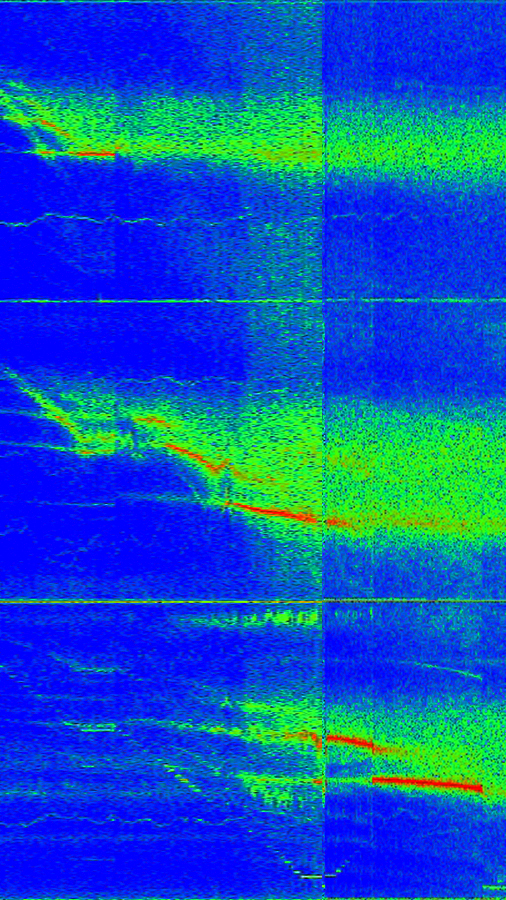A plot of the measured magnetic field of one of our wave modes. We're measuring the component of the field along a cylindrical radius (i.e., pointing outward from the axis of rotation) over the whole sphere. Theta and phi are the polar and azimuthal coordinate, respectively.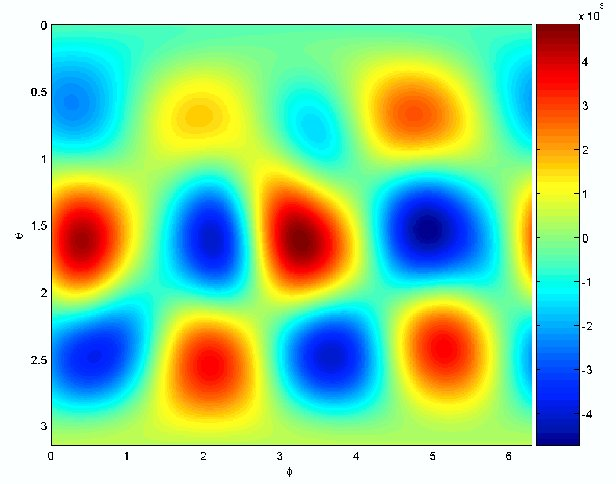Theoretical calculation of the velocity and kinetic energy fields of the inertial wave mode that we believe is producing the induction shown in the second figure.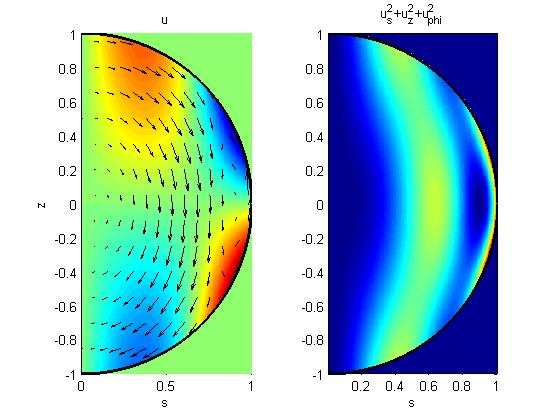Demonstration of the sensitive dependance on initial conditions in a Rayleigh-Benard convection simulation. The fluid is in a state of spiral defect chaos. Plotted is the difference between two perturbed initial conditions.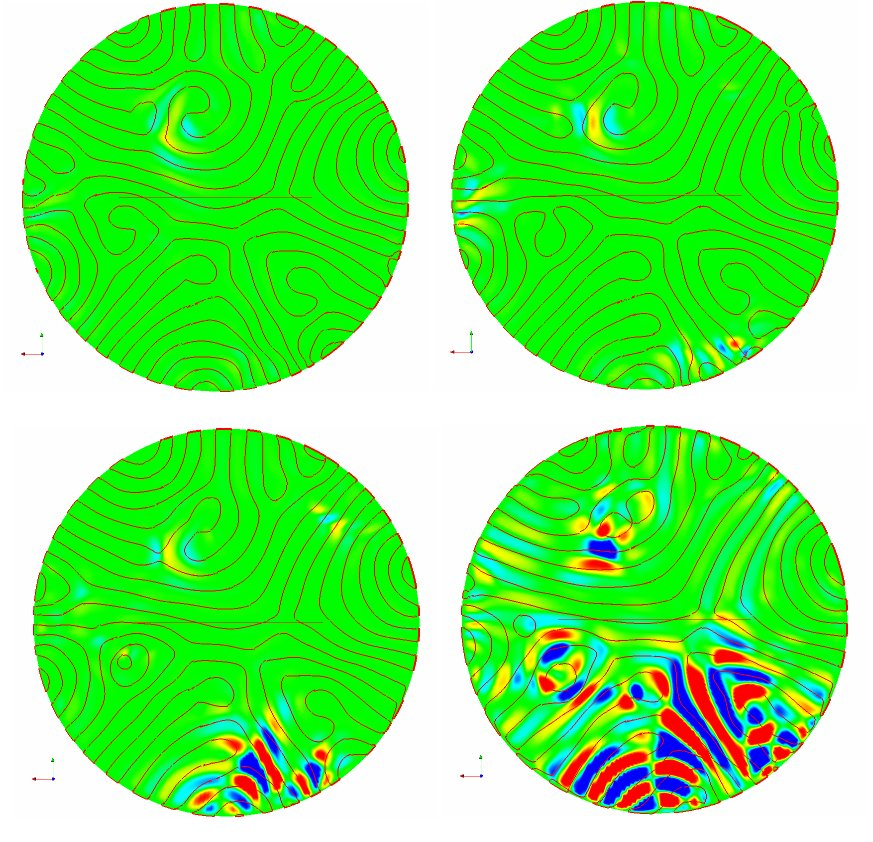A vector field of the mean flow in a Rayleigh-Benard convection simulation. The collors indicate regions of hot (red) and cold (blue) fluid.